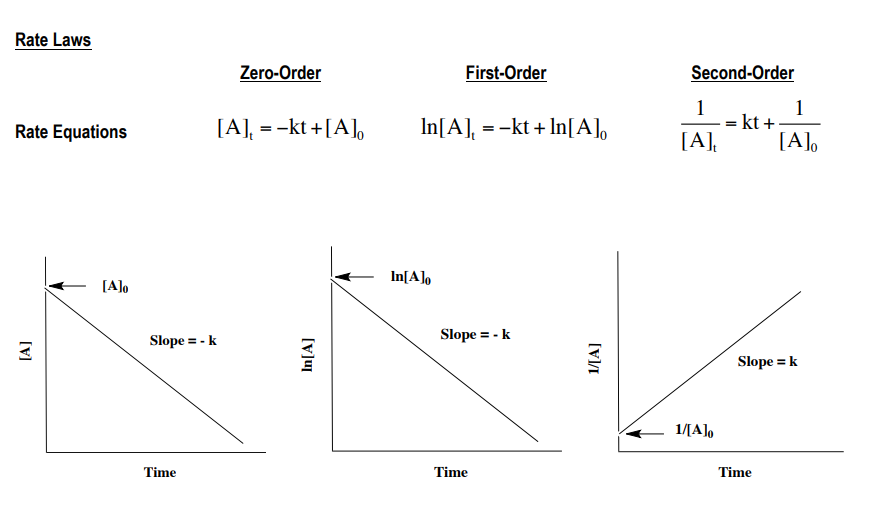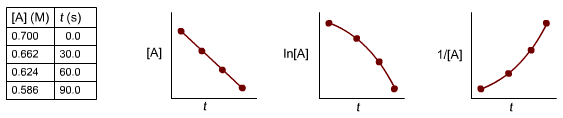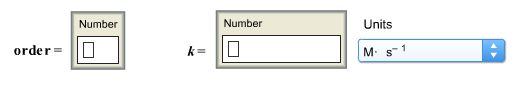# Problem: For A → products, time and concentration data were collected and plotted as shown below.Determine the reaction order, the rate constant, and the units of the rate constant.

###### FREE Expert Solution88% (493 ratings)###### Problem Details

For A → products, time and concentration data were collected and plotted as shown below.Determine the reaction order, the rate constant, and the units of the rate constant.Frequently Asked Questions

What scientific concept do you need to know in order to solve this problem?

Our tutors have indicated that to solve this problem you will need to apply the Integrated Rate Law concept. You can view video lessons to learn Integrated Rate Law. Or if you need more Integrated Rate Law practice, you can also practice Integrated Rate Law practice problems.